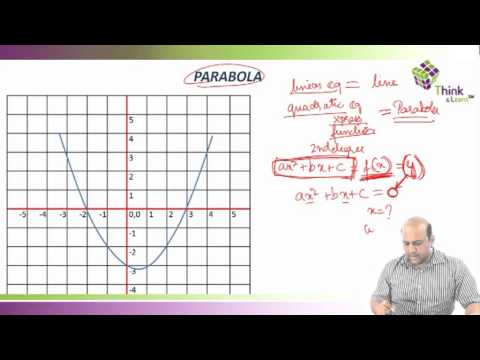# How to crack GMAT Quantitative Ability Questions

The CAT Quant section basically tests your numerical ability and mathematical skills. The section covers Arithmetic, Algebra and Geometry and questions predominantly belongs to what is taught to students of tenth standard. So, instead of panicking, you should concentrate on brushing up the basic concepts and tricks to solve CAT quant questions confidently. Crack CAT Quantitative Ability questions in Byju’s way!In this video, we are explaining about quadratic equation. A quadratic equation is a second-order polynomial equation in a single variable since it is a second-order polynomial equation, the fundamental theorem of algebra guarantees that it has two solutions. These solutions may be both real, or both complex. Want to know more about our way of training?

For more details SMS your Name, Contact No. and Email ID to +918884544444  and to get a 7 days free tablet training program!